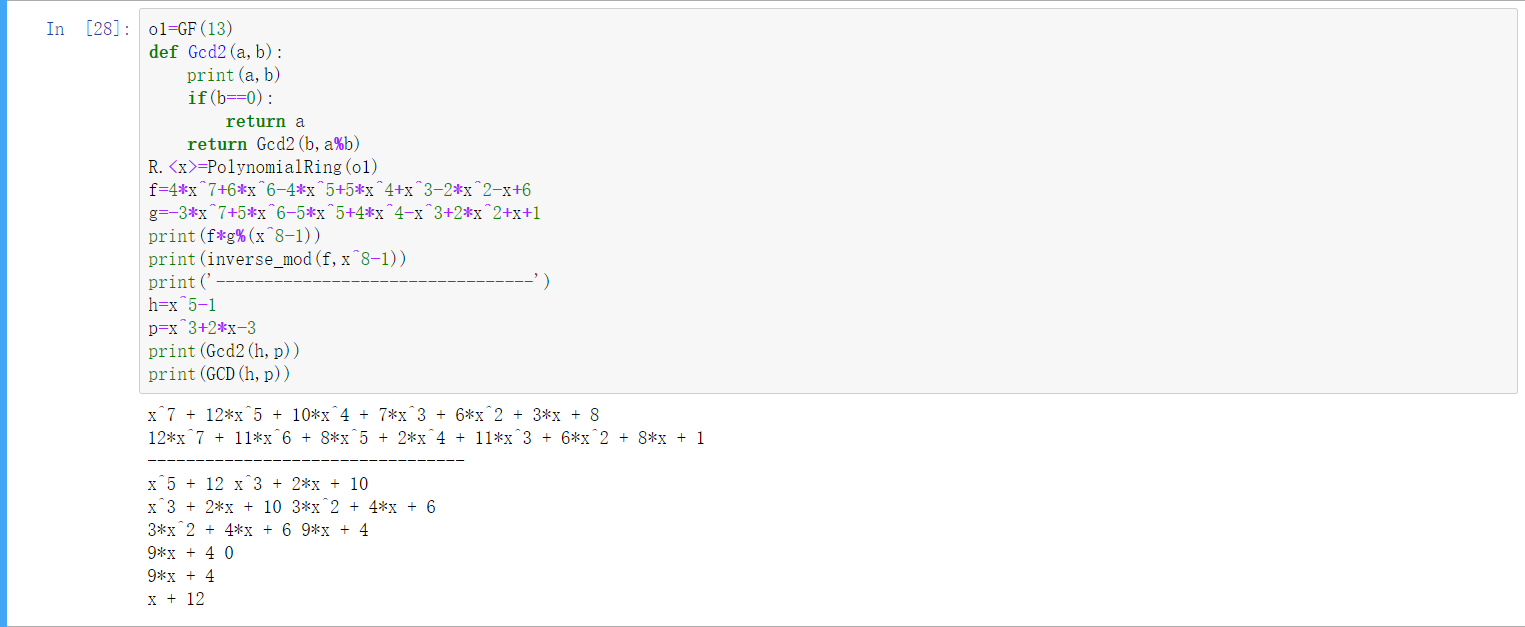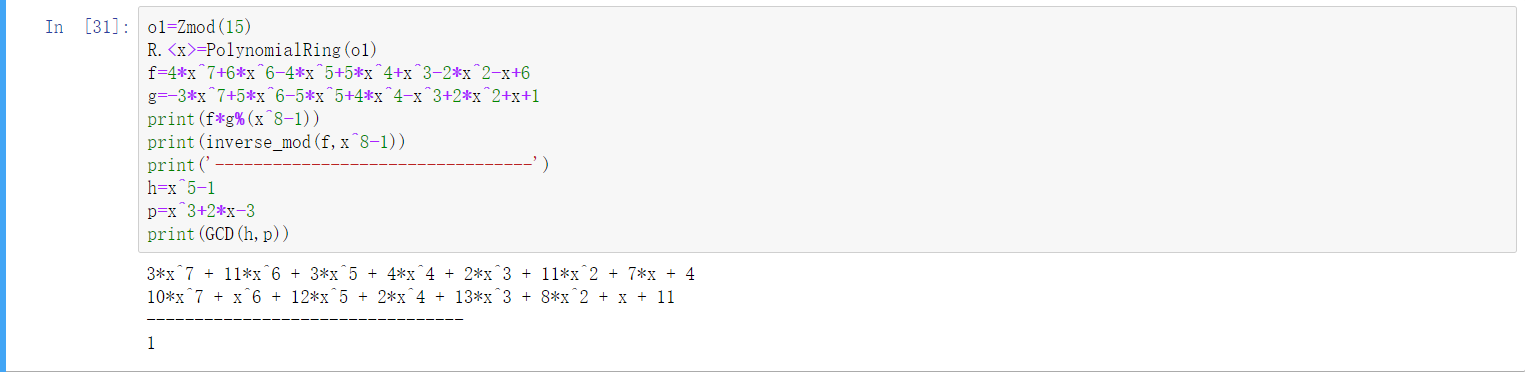# huangx607087学习格密码的笔记3

## 6.Babai算法和寻找好的基底解决apprCVP问题

$$\vec w=\sum_{i=1}^n b_i\vec v_i,b_i \in R$$

## 7.基于困难格问题的密码

[Translated by baidu translation]

Ajtai-Dwork系统特别有趣，因为Ajtai和Dwork表明，除非在多项式时间内解决最坏情况下的晶格问题，否则它们的系统是可证明的安全的。 抵消这一重要的理论结果是密钥大小被证明是$O(N^4)$的实际限制，这导致了巨大的密钥。 阮和斯特恩随后表明，Ajtai-Dwork系统的任何实际和有效的实现都是不安全的。

## 8.GGH公钥密码系统

### 8x01 加密过程

Alice首先选取一组线性无关的向量组$\vec v_1,\vec v_2,…,\vec v_n$，它们之间是相当正交的。这样做的一个方法是确定一个参数$d>0$，然后在$-d$与$d$之间随机选择基底向量$\vec v$的坐标。也可以计算它的Hadamard比率 来看看它们是否相当正交。确定好向量之后，就构建一个以$\vec v_1$到$\vec v_n$为基底的格$L$和由这些（行）向量构建的一个矩阵$V$。这些向量也将成为Alice的私钥。

Alice然后选择一个$n×n$的矩阵$U$，满足$U$中所有元素均为整数且$\det ^2 U=1$。然后计算$W=UV$，并将$W$中所有的行向量作为公钥。

Alice解密时也很直接，她可以使用Babai算法计算与$\vec c$最接近的格向量$\vec d$，然后计算$\vec dW^{-1}$就可以恢复明文$\vec m$

Alice构建如下矩阵$U$（其行列式为$-1$）,然后计算$W=UV$如下图### 8x02 附注

1.我们观察到GGH是概率密码系统的一个例子，因为由于随机扰动r的选择，单个明文导致许多不同的密文。如果Bob使用不同的随机扰动两次发送相同的消息，或者使用相同的随机扰动发送不同的消息，这将导致潜在的危险。 因此，在实践中，随机扰动r是通过将哈希函数应用于明文$m$来确定的。

2.另一个版本的GGH逆转了$m$和$r$的角色，因此密文具有形式$c=\vec rW+\vec m$。Alice通过计算最接近$c$的格向量找到$\vec rW$，然后她将明文恢复为$\vec m=\vec c−\vec rW$。

## 9.卷积多项式环

### 9x02 无模数情况下的计算法则

$$f(x)+g(x)=\sum_{i=0}^{n-1}(a_i+b_i)x^i$$

$$f(x)g(x)=\sum_{i=0}^{n-1} \sum_{j+k\equiv i \pmod n} a_jb_k$$

### 9x03 有模数情况下的计算法则

$$h(x)=4x^4-7x^3-7x^2-6x+5$$

### 9x04 素数域中的乘法运算Update 7.29 增加了具体实现方法：

$$f(x)F(x)\equiv 1 \pmod {p^i}$$

$$G(x)=(2-f(x)F(x))F(x)$$

目录